Next: The Goldwasser-Micali encryption system Up: Blair's Cryptography Notes Previous: Analysis of the Rabin   Contents

# Probabilistic Encryption

[References to ``the paper'' in this section are to ``Probabilistic Encryption,'' in Journal of Computer & System Sciences 28, pp. 270-299. I have also used Primality and Cryptography, by E. Kranakis]

So far, the public key systems have been functions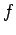such that the message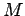presumably cannot be computed from the encoding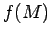. A further concern arises as to whether, even if the adversary cannot identifyexactly, he may be able to obtain some partial information about, for example tell whetheris an even number, a square, a power of 2, etc.

An extreme case of this would be a scenario in which the adversary knows the message is one of two possibilities,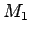or. Since we have been assuming that the functionis easy to calculate, all the adversary needs to do is compareand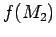with the ciphertext.

Probabilistic encryption is a system designed to avoid these problems. Instead ofbeing a single number, the calculation ofinvolves the sender doing some things randomly during the calculation, so thathas many different encryptions. Indeed, the probability should be very close to 1 that if the same message is sent twice, the encryptions should be different.

SubsectionsNext: The Goldwasser-Micali encryption system Up: Blair's Cryptography Notes Previous: Analysis of the Rabin   Contents
Translated from LaTeX by Scott Sutherland
2002-12-14